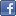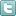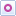EE Times-India > T&M

T&M# Fix distortion using internal noise as test signal

Posted: 23 Dec 2015Print Version

Keywords:distortion  postprocessing  calibration  ﬁltering  amplifier

This article continues the theme of my previous one: distortion correction. That article addressed the case of steady-state distortion minimisation. This time, we look at an idea that addresses the real-time version of the problem which, being more general, tends to be harder.

One general idea to compensate for distortion is to look at the postprocessing stage, where by inverting the transfer function of the device or system under test, we can effectively cancel the effects of distortion. This usually makes the approximation that the transfer function itself is acceptably steady with time or with input signal variation. In order to do this, the transfer function must be accurately measured, and under the above approximation this may be measured very accurately by taking the system offline, and applying to it a reference signal which may be a ramp, a sinusoid, or a noise signal. However, what if it is not easy or desirable to take system offline for the purpose of calibration? This question was addressed by L.J. Gunn, A. Allison and D. Abbott of the School of Electrical and Electronic Engineering, The University of Adelaide, in a paper titled "Identification of static distortion by noise measurement" published in Electronics Letters, Vol. 49 No. 21 pp. 1321–1323, October 2013.

The method
The authors' idea hinges on the premise that the internal noise in an electronic circuit can provide sufﬁcient information to characterise its static non-linearity without knowledge of the input. The model used for the noise at the input is one of white noise whose variance is a constant σ2. If the signal is denoted by x(t) and the noise by N(t), then at the input we have Z(t) = x(t) + N(t) corresponding to which the system produces an output Y(t) = f(Z(t)) = f(x(t) + N(t)). If the system is largely linear, we can linearise the transfer function around x(t), giving us:(where the derivative is with respect to x(t), not with respect to time). Note that the function f´(x(t)) is the dynamic gain of the system. The objective now is to get to an estimate of x(t), the original undistorted signal. Taking the variance of both sides over a small interval of time so that x(t) remains almost constant, we get:Note that this result is quite intuitive. All we are saying is that if the standard deviation of the signal x(t) is zero, the dynamic gain of the system is the ratio of the standard deviation of the output to the standard deviation of the noise signal. Now if we also have an estimate of f(x(t)), we could complete the picture. The signal x(t) is assumed to be band-limited and relatively slow-varying, so f(x(t)) can be estimated by using a lowpass filter. Now that we have f(x(t)) as well as f´(x(t)), we get:which is the estimate of x(t) we were after. The constant of integration is just the offset of the input signal. The above integrals are computed numerically by measuring f(x(t)) and f'(x(t)). This allows us to compute a compensation factor. In essence then, the method uses a low-frequency band-limited signal with the system's internal white noise superimposed on it (rather than an external calibration signal) to derive the compensation factor.

 Related Articles Editor's Choice
Comment on "Fix distortion using internal noise ..."

Top Ranked Articles

WebinarsVisit Asia Webinars to learn about the latest in technology and get practical design tips.

Search EE Times India
Services

Go to topConnect on FacebookFollow us on TwitterFollow us on Orkut
﻿

Back to Top